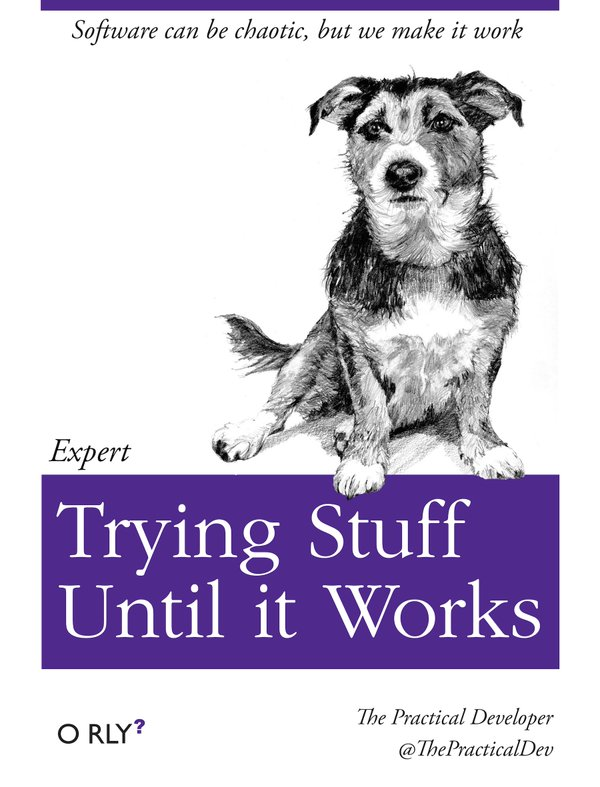# SICP 1.2.6 Primes

Published: 2020-06-21T16:22:37.000Z

A little bit of number theory.

## Exercise 1.21: Smallest divisors

This is so trivial, so I'll try to rewrite example, because it's just copypaste otherwise:

``````(define (divisor? d n) (= (remainder n d) 0))
(define (smallest-divisor n)
(define (find-divisor n d)
(cond ((> (sqr d) n) n)
((divisor? d n) d)
(else (find-divisor n (+ d 1)))
)

)
(find-divisor n 2)
)``````
``````1 ]=> (smallest-divisor 199)

;Value: 199

1 ]=> (smallest-divisor 1999)

;Value: 1999

1 ]=> (smallest-divisor 19999)

;Value: 7``````

Well, that was unexpected. :)

## Exercise 1.22:

``````(define (prime? n)
(= n (smallest-divisor n)))
(define (next-prime n)
(if (prime? n)
n
(next-prime (+ n 2))
)
)
(define (timed-prime-test n)
(define (start-prime-test n start-time)
(display "next prime after ") (display n)
(display ": ") (display (next-prime n)) (newline)
(display "Time: ") (display (- (runtime) start-time))
(newline)
)
(start-prime-test n (runtime))
)
(timed-prime-test 10000000001)
; next prime after 10000000001: 10000000019
; Time: .19999999999998863
(timed-prime-test 1000000000001)
; next prime after 1000000000001: 1000000000039
; Time: 1.3700000000000045``````

Seems to take 10 times more time for 100 times bigger number.

## Exercise 1.23

``````(define (next n)
(if (= n 2) 3 (+ n 2))
)
(define (smallest-divisor n)
(define (find-divisor n d)
(cond ((> (sqr d) n) n)
((divisor? d n) d)
(else (find-divisor n (next d)))
)

)
(find-divisor n 2)
)

(timed-prime-test 10000000001)
;Time: .12000000000000455

(timed-prime-test 1000000000001)
; Time: .8000000000000114``````

A little bit less than twice as fast. I assume this is because calling next and doing comparisons inside it takes more time than just (+ n 1)

## Exercise 1.24

``````(define (expmod base exp m)
(cond ((= exp 0) 1)
((even? exp)
(remainder (square (expmod base (/ exp 2) m))
m))
(else
(remainder (* base (expmod base (- exp 1) m))
m))))
(define (fermat-test n)
(define (try-it a)
(= (expmod a n n) a))
(try-it (+ 1 (random (- n 1)))))
(define (fast-prime? n times)
(cond ((= times 0) true)
((fermat-test n) (fast-prime? n (- times 1)))
(else false)))
(define (next-prime n)
(if (fast-prime? n 10)
n
(next-prime (+ n 2))
)
)

(timed-prime-test 1000000000001)
; next prime after 1000000000001: 1000000000039
; Time: 9.999999999990905e-3

(timed-prime-test 10000000000000000000001)
; next prime after 10000000000000000000001: 10000000000000000000009
; Time: 9.999999999990905e-3``````

Time seems to not change at all (but of course first search checked more numbers).

## Exercise 1.25

That would slow down our computations tremendously, because we would need to multiply huge numbers. With millions of digits, which would take megabytes of memory.

## Exercise 1.26

In correct `expmod`, every time `expmod` is recursively called with half of `exp` argument, it is called once.

In Louis Reasoner implementation, when `expmod` is called with halved `exp` argument, it is called twice, so halving the argument is compensated by doubling the tree of recursion, and that's why number of calls to `expmod` is proportional to the argument.

## Exercise 1.27

``````(define (full-fermat n)
(define (f-prime? a)
(= (expmod a n n) a)
)
(define (iter a)
(if (= a n)
#t ; test passed
(if (f-prime? a)
(iter (+ a 1))
#f; test failed
)
)
)
(iter 2)
)

(full-fermat 13); #t
(full-fermat 15); #f
(full-fermat 561); #t
(full-fermat 1105); #t
(full-fermat 1729); #t``````

## Exercise 1.28: Miller-Rabin test

``````(define (!= a b) (not (= a b))) ; I need some Scheme reference, really. How this is not built-in?

(define (report n m)
(display n)
(display " is a nontrivial square root of 1 modulo ")
(display m)
(newline)
0
)
(define (expmod base exp m)
(define (squaremod-signal n)
(define (is-root? sq)
(if (and  (= sq 1) (!= n 1) (!= n (- m 1)))
(report n m)
sq
)
)
(is-root? (remainder (square n) m))
)
(cond ((= exp 0) 1)
((even? exp)
(squaremod-signal (expmod base (/ exp 2) m)))
(else
(remainder (* base (expmod base (- exp 1) m))
m))))
(define (fast-prime? n times)
(cond ((= times 0) true)
((miller-rabin-test n) (fast-prime? n (- times 1)))
(else false)))
(define (miller-rabin-test n)
(define (try-it a)
(!= (expmod a (- n 1) n) 0)
)
(if (even? n)
#f
(try-it (+ 1 (random (- n 1))))
)
)
(fast-prime? 1009 30)
(fast-prime? 13 30)
(fast-prime? 6601 30)``````

Whew! How this even work - no idea, but somehow it does. Thankfully I'm good in this technique: